# Precision-Recall¶

Example of Precision-Recall metric to evaluate classifier output quality.

Precision-Recall is a useful measure of success of prediction when the classes are very imbalanced. In information retrieval, precision is a measure of result relevancy, while recall is a measure of how many truly relevant results are returned.

The precision-recall curve shows the tradeoff between precision and recall for different threshold. A high area under the curve represents both high recall and high precision, where high precision relates to a low false positive rate, and high recall relates to a low false negative rate. High scores for both show that the classifier is returning accurate results (high precision), as well as returning a majority of all positive results (high recall).

A system with high recall but low precision returns many results, but most of its predicted labels are incorrect when compared to the training labels. A system with high precision but low recall is just the opposite, returning very few results, but most of its predicted labels are correct when compared to the training labels. An ideal system with high precision and high recall will return many results, with all results labeled correctly.

Precision (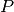) is defined as the number of true positives (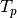) over the number of true positives plus the number of false positives (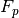).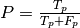Recall (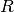) is defined as the number of true positives () over the number of true positives plus the number of false negatives (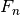).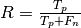These quantities are also related to the (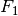) score, which is defined as the harmonic mean of precision and recall.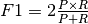Note that the precision may not decrease with recall. The definition of precision (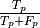) shows that lowering the threshold of a classifier may increase the denominator, by increasing the number of results returned. If the threshold was previously set too high, the new results may all be true positives, which will increase precision. If the previous threshold was about right or too low, further lowering the threshold will introduce false positives, decreasing precision.

Recall is defined as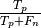, where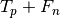does not depend on the classifier threshold. This means that lowering the classifier threshold may increase recall, by increasing the number of true positive results. It is also possible that lowering the threshold may leave recall unchanged, while the precision fluctuates.

The relationship between recall and precision can be observed in the stairstep area of the plot - at the edges of these steps a small change in the threshold considerably reduces precision, with only a minor gain in recall.

Average precision (AP) summarizes such a plot as the weighted mean of precisions achieved at each threshold, with the increase in recall from the previous threshold used as the weight: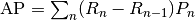where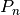and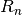are the precision and recall at the nth threshold. A pair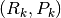is referred to as an operating point.

AP and the trapezoidal area under the operating points (sklearn.metrics.auc) are common ways to summarize a precision-recall curve that lead to different results. Read more in the User Guide.

Precision-recall curves are typically used in binary classification to study the output of a classifier. In order to extend the precision-recall curve and average precision to multi-class or multi-label classification, it is necessary to binarize the output. One curve can be drawn per label, but one can also draw a precision-recall curve by considering each element of the label indicator matrix as a binary prediction (micro-averaging).

from __future__ import print_function


## In binary classification settings¶

### Create simple data¶

Try to differentiate the two first classes of the iris data

from sklearn import svm, datasets
from sklearn.model_selection import train_test_split
import numpy as np

X = iris.data
y = iris.target

random_state = np.random.RandomState(0)
n_samples, n_features = X.shape
X = np.c_[X, random_state.randn(n_samples, 200 * n_features)]

# Limit to the two first classes, and split into training and test
X_train, X_test, y_train, y_test = train_test_split(X[y < 2], y[y < 2],
test_size=.5,
random_state=random_state)

# Create a simple classifier
classifier = svm.LinearSVC(random_state=random_state)
classifier.fit(X_train, y_train)
y_score = classifier.decision_function(X_test)


### Compute the average precision score¶

from sklearn.metrics import average_precision_score
average_precision = average_precision_score(y_test, y_score)

print('Average precision-recall score: {0:0.2f}'.format(
average_precision))


Out:

Average precision-recall score: 0.88


### Plot the Precision-Recall curve¶

from sklearn.metrics import precision_recall_curve
import matplotlib.pyplot as plt

precision, recall, _ = precision_recall_curve(y_test, y_score)

plt.step(recall, precision, color='b', alpha=0.2,
where='post')
plt.fill_between(recall, precision, step='post', alpha=0.2,
color='b')

plt.xlabel('Recall')
plt.ylabel('Precision')
plt.ylim([0.0, 1.05])
plt.xlim([0.0, 1.0])
plt.title('2-class Precision-Recall curve: AP={0:0.2f}'.format(
average_precision))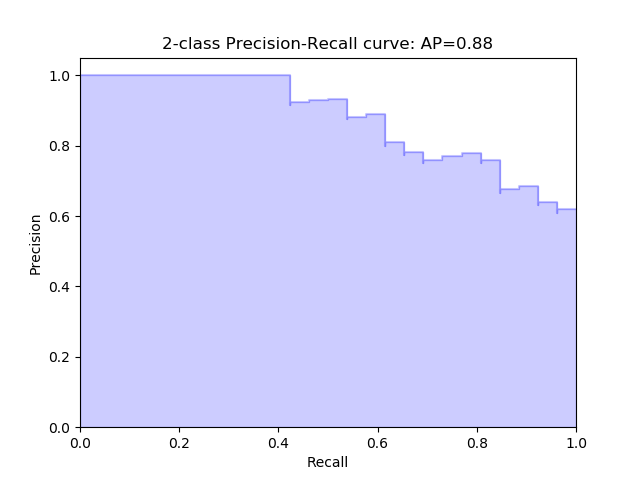## In multi-label settings¶

### Create multi-label data, fit, and predict¶

We create a multi-label dataset, to illustrate the precision-recall in multi-label settings

from sklearn.preprocessing import label_binarize

# Use label_binarize to be multi-label like settings
Y = label_binarize(y, classes=[0, 1, 2])
n_classes = Y.shape

# Split into training and test
X_train, X_test, Y_train, Y_test = train_test_split(X, Y, test_size=.5,
random_state=random_state)

# We use OneVsRestClassifier for multi-label prediction
from sklearn.multiclass import OneVsRestClassifier

# Run classifier
classifier = OneVsRestClassifier(svm.LinearSVC(random_state=random_state))
classifier.fit(X_train, Y_train)
y_score = classifier.decision_function(X_test)


### The average precision score in multi-label settings¶

from sklearn.metrics import precision_recall_curve
from sklearn.metrics import average_precision_score

# For each class
precision = dict()
recall = dict()
average_precision = dict()
for i in range(n_classes):
precision[i], recall[i], _ = precision_recall_curve(Y_test[:, i],
y_score[:, i])
average_precision[i] = average_precision_score(Y_test[:, i], y_score[:, i])

# A "micro-average": quantifying score on all classes jointly
precision["micro"], recall["micro"], _ = precision_recall_curve(Y_test.ravel(),
y_score.ravel())
average_precision["micro"] = average_precision_score(Y_test, y_score,
average="micro")
print('Average precision score, micro-averaged over all classes: {0:0.2f}'
.format(average_precision["micro"]))


Out:

Average precision score, micro-averaged over all classes: 0.43


### Plot the micro-averaged Precision-Recall curve¶

plt.figure()
plt.step(recall['micro'], precision['micro'], color='b', alpha=0.2,
where='post')
plt.fill_between(recall["micro"], precision["micro"], step='post', alpha=0.2,
color='b')

plt.xlabel('Recall')
plt.ylabel('Precision')
plt.ylim([0.0, 1.05])
plt.xlim([0.0, 1.0])
plt.title(
'Average precision score, micro-averaged over all classes: AP={0:0.2f}'
.format(average_precision["micro"]))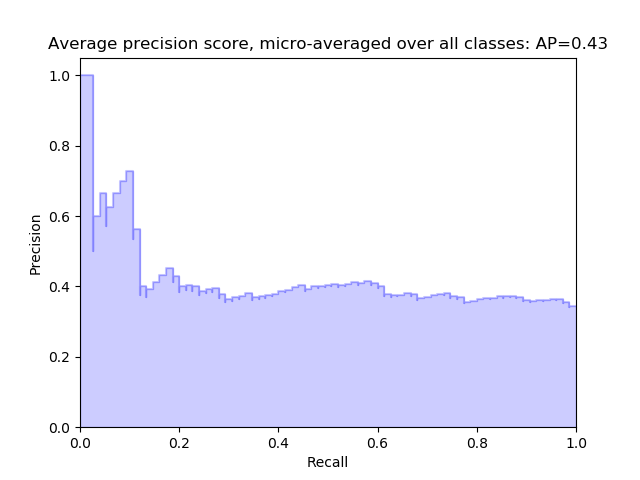### Plot Precision-Recall curve for each class and iso-f1 curves¶

from itertools import cycle
# setup plot details
colors = cycle(['navy', 'turquoise', 'darkorange', 'cornflowerblue', 'teal'])

plt.figure(figsize=(7, 8))
f_scores = np.linspace(0.2, 0.8, num=4)
lines = []
labels = []
for f_score in f_scores:
x = np.linspace(0.01, 1)
y = f_score * x / (2 * x - f_score)
l, = plt.plot(x[y >= 0], y[y >= 0], color='gray', alpha=0.2)
plt.annotate('f1={0:0.1f}'.format(f_score), xy=(0.9, y + 0.02))

lines.append(l)
labels.append('iso-f1 curves')
l, = plt.plot(recall["micro"], precision["micro"], color='gold', lw=2)
lines.append(l)
labels.append('micro-average Precision-recall (area = {0:0.2f})'
''.format(average_precision["micro"]))

for i, color in zip(range(n_classes), colors):
l, = plt.plot(recall[i], precision[i], color=color, lw=2)
lines.append(l)
labels.append('Precision-recall for class {0} (area = {1:0.2f})'
''.format(i, average_precision[i]))

fig = plt.gcf()
plt.xlim([0.0, 1.0])
plt.ylim([0.0, 1.05])
plt.xlabel('Recall')
plt.ylabel('Precision')
plt.title('Extension of Precision-Recall curve to multi-class')
plt.legend(lines, labels, loc=(0, -.38), prop=dict(size=14))

plt.show()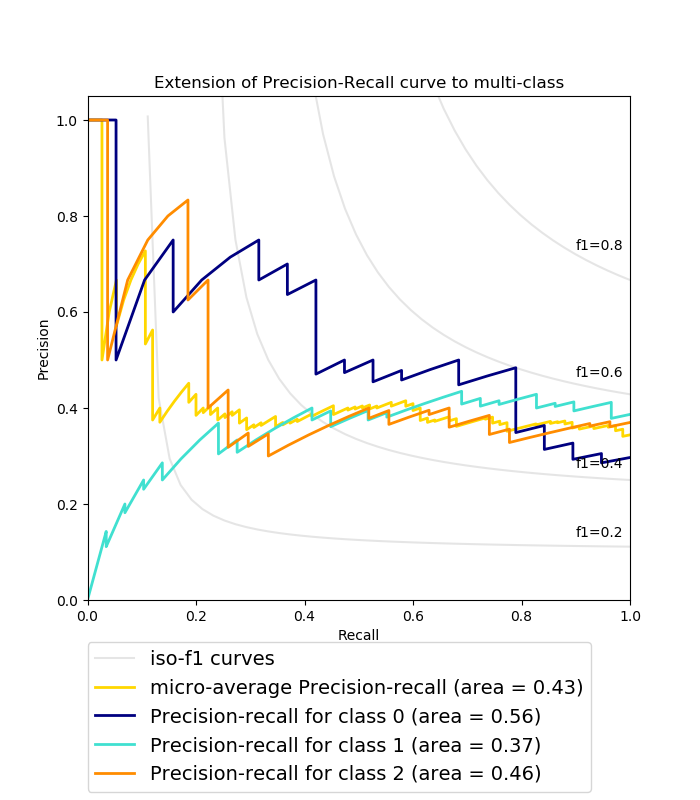Total running time of the script: ( 0 minutes 0.128 seconds)

Generated by Sphinx-Gallery